On Jan 19, schools, going, to open, for, ssc, and hsc, tamilnadu,

COVID-19 தொற்றுநோய்க்கு மத்தியில் முன்னெச்சரிக்கை நடவடிக்கையாக 2020 மார்ச்சில் பள்ளிகள் மூடப்பட்டன.

ஜனவரி 19 ஆம் தேதி தமிழகம் முழுவதும் உள்ள பள்ளிகள் 10 மற்றும் 12 ஆம் வகுப்பு மாணவர்களுக்கு மீண்டும் திறக்கப்படும் என்று தமிழக அரசு செவ்வாய்க்கிழமை அறிவித்தது. இந்த மாணவர்களுக்கான விடுதிகளும் ஜனவரி 19 ஆம் தேதி மீண்டும் திறக்க அனுமதிக்கப்பட்டுள்ளன.

தமிழக முதல்வர் வெளியிட்டுள்ள செய்திக்குறிப்பில், பெற்றோர் உட்பட அனைத்து தரப்பினருடனும் கலந்தாலோசித்த பின்னர் இந்த முடிவு எடுக்கப்பட்டுள்ளது. வாரியத் தேர்வுகளுக்கு முன்னதாக, 10 மற்றும் 12 வகுப்புகளுக்கான பள்ளிகளை மீண்டும் திறப்பது தொடர்பாக ஜனவரி 6 முதல் 8 வரை பெற்றோர்களுடன் ஆலோசனை அமர்வுகளை நடத்த மாநில அரசு உத்தரவிட்டது. “95% பள்ளிகள் இந்த மாணவர்களுக்கான பள்ளிகளை மீண்டும் திறக்க பெரும்பாலான பெற்றோர்கள் ஒப்புக் கொண்டதாக அறிக்கைகளை சமர்ப்பித்துள்ளன” என்று அந்த அறிக்கையில் தெரிவிக்கப்பட்டுள்ளது. மாணவர்களின் கல்வி நலனைக் கருத்தில் கொண்டு, ஜனவரி 19 ஆம் தேதி இந்த மாணவர்களுக்கான பள்ளிகளை மீண்டும் திறக்க அரசாங்கம் அனுமதி வழங்கியுள்ளது. “ஒவ்வொரு வகுப்பிலும் 25 மாணவர்கள் மட்டுமே இருக்க வேண்டும், மேலும் பள்ளிகள் வழங்கிய நிலையான இயக்க முறைகளைப் பின்பற்ற வேண்டும். மாநில அரசு, ”என்று அந்த அறிக்கை மேலும் கூறியுள்ளது.

கோவிட் -19 தொற்றுநோயால் 2020 மார்ச் கடைசி வாரத்தில் இருந்து தமிழ்நாட்டின் பள்ளிகள் மூடப்பட்டுள்ளன. போர்டு தேர்வுகள் மட்டுமே மாணவர்களுக்கு நடத்தப்பட்டன, மற்ற அனைத்து மாணவர்களும் அடுத்த வகுப்பிற்கு தானாக பதவி உயர்வு அறிவிக்கப்பட்டனர்.

தீபாவளிக்குப் பிறகு பள்ளிகளைத் திறக்க அரசாங்கம் திட்டமிட்டிருந்தது, இருப்பினும், கோவிட் -19 நிலைமை இன்னும் மாநிலத்தில் கட்டுப்பாட்டில் இல்லாததால் இந்த நடவடிக்கை பல்வேறு தரப்பினரிடமிருந்தும் பரவலான விமர்சனங்களைப் பெற்றது. இதைத் தொடர்ந்து, மாநில அரசு தனது அறிவிப்பை திரும்பப் பெற்றது.

தமிழகம், திங்களன்று, கோவிட் -19 புதிய 682 வழக்குகள் பதிவாகியுள்ளன. COVID-19 க்கு தற்போது 6,971 பேர் சிகிச்சை பெற்று வருகின்றனர். COVID-19 காரணமாக திங்களன்று 6 பேர் இறந்ததாக தமிழகம் தெரிவித்துள்ளது, மொத்த இறப்பு எண்ணிக்கை 12,228 ஆக உள்ளது. திங்களன்று 869 பேர் தொற்றுநோயிலிருந்து மீட்கப்பட்டதாகவும், திங்களன்று நிலவரப்படி COVID-19 இலிருந்து மீட்கப்பட்ட நோயாளிகளின் எண்ணிக்கை 8,07,744 என்றும் தெரிவிக்கப்பட்டுள்ளது.

Tamilnadu, Samacheer, Kalvi, 10th, sslc, Maths, Solutions, Chapter 5, Coordinate Geometry, Unit Exercise 5,

Question 1.
PQRS is a rectangle formed by joining the points P(- 1, – 1), Q(- 1, 4) , R(5, 4) and S (5, – 1). A, B, C and D are the mid-points of PQ, QR, RS and SP respectively. Is the quadrilateral ABCD a square, a rectangle or a rhombus? Justify your answer.

Mid point of a line = ([Math Processing Error])
Mid point of PQ (A) = ([Math Processing Error],[Math Processing Error])
= ([Math Processing Error],[Math Processing Error]) = (-1,[Math Processing Error])
Mid point of QR (B) = ([Math Processing Error],[Math Processing Error]) = ([Math Processing Error],[Math Processing Error]) = (2,4)
Mid point of RS (C) = ([Math Processing Error],[Math Processing Error]) = ([Math Processing Error],[Math Processing Error]) = (5,[Math Processing Error])
Mid point of PS (D) = ([Math Processing Error],[Math Processing Error]) = ([Math Processing Error],[Math Processing Error]) = (2,-1)

img 355
AB = BC = CD = AD = [Math Processing Error]
Since all the four sides are equal,
∴ ABCD is a rhombus.

Question 2.
The area of a triangle is 5 sq. units. Two of its vertices are (2,1) and (3, -2). The third vertex is (x, y) where y = x + 3 . Find the coordinates of the third vertex.
Let the vertices A(2,1), B(3, – 2) and C(x, y)
Area of a triangle = 5 sq. unit

[Math Processing Error] [x1y2 + x2y3 + x3y1– (x2y1 + x3y2 + x1y3)] = 5
[Math Processing Error] [-4 + 3y + x – (3 – 2x + 2y)] = 5
-4 + 3y + x – 3 + 2x – 2y = 10
3x + y – 7 = 10
3x + y = 17 ……(1)
Given y = x + 3
Substitute the value ofy = x + 3 in (1)
3x + x + 3 = 17
4x = 17 – 3
4x = 14
x = [Math Processing Error] = [Math Processing Error]
Substitute the value of x in y = x + 3
y = [Math Processing Error] + 3 ⇒ y = [Math Processing Error] = [Math Processing Error]
∴ The coordinates of the third vertex is ([Math Processing Error],[Math Processing Error])

www.asterclasses.com

Question 3.
Find the area of a triangle formed by the lines 3x + y – 2 = 0, 5x + 2y – 3 = 0 and 2x – y – 3 = 0
3x + y = 2 ……..(1)
5x + 2y = 3 ………(2)
2x – y = 3 ……….(3)
Solve (1) and (2) to get the vertices Bp

Substitute the value of x = 1 in (1)
3(1) + y = 2
y = 2 – 3 = – 1
The point B is (1, – 1)
Solve (2) and (3) to get the vertices C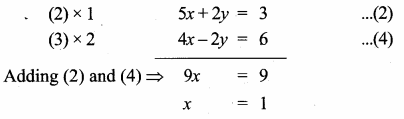Substitute the value of x = 1 in (3)
2(1) – y = 3 ⇒ -y = 3 – 2
– y = 1 ⇒ y = – 1
The point C is (1, – 1)
Solve (1) and (3) to get the vertices ASubstitute the value of x = 1 in (1)
3(1) + y = 2
y = 2 – 3 = -1
The point A is (1, – 1)
The points A (1, – 1), B (1, -1), C(1, -1)
Area of ∆ABC = [Math Processing Error]

[x1y2 + x2y3 + x3y1 – (x2y1 + x3y2 + x1y3)]

Area of the triangle = 0 sq. units.
Note: All the three vertices are equal, all the point lies in a same points.

www.asterclasses.com

Question 4.
If vertices of a quadrilateral are at A(- 5, 7), B(- 4, k), C(- 1, – 6) and D(4, 5) and its area is 72 sq.units. Find the value of k.

Area of the quadrilateral ABCD = 72 sq. units.
[Math Processing Error] [(x1y2 + x2y3 + x3y4 + x4y1) – (x2y1 + x3y2 + x4y3 + x1y4)] = 72

-5k + 24 – 5 + 28 – (- 28 – K – 24 – 25) = 144
– 5k + 47 – k – 77 = 144
– 5k + 47 + k + 77 = 144
– 4k + 124 = 144
-4k = 144 – 124
– 4k = 20
k = -5
The value of k = – 5

www.asterclasses.com

Question 5.
Without using distance formula, show that the points (-2,-1), (4,0), (3,3) and (-3,2) are vertices of a parallelogram.
The vertices A(-2, -1), B(4, 0), C(3, 3) and D(- 3, 2)

Slope of a line = [Math Processing Error]
Slope of AB = [Math Processing Error] = [Math Processing Error]
Slope of BC = [Math Processing Error] = [Math Processing Error] = -3
Slope of CD = [Math Processing Error] = [Math Processing Error] = [Math Processing Error]
Slope of AD = [Math Processing Error] = [Math Processing Error] = -3
Slope of AB = Slope of CD = [Math Processing Error]
∴ AB || CD ……(1)
Slope of BC = Slope of AD = -3
From (1) and (2) we get ABCD is a parallelogram.

Question 6.
Find the equations of the lines, whose sum and product of intercepts are 1 and – 6 respectively.
Let the “x” intercept be “a”
y intercept = 1 – a (sum of the intercept is 1)
Product of the intercept = – 6
a (1 – a) = – 6 ⇒ a – a2 = – 6
– a2 + a + 6 = 0 ⇒ a2 – a – 6 = 0
(a – 3) (a + 2) = 0 ⇒ a – 3 = 0 (or) a + 2 = 0
a = 3 (or) a = -2
When a = 3
x – intercept = 3
y – intercept = 1 – 3 = – 2
Equation of a line is
[Math Processing Error] + [Math Processing Error] = 1
[Math Processing Error] + [Math Processing Error] = 1
[Math Processing Error] – [Math Processing Error] = 1
2x – 3y = 6
2x – 3y – 6 = 0

When a =-2
x – intercept = -2
y – intercept = 1 – (- 2) = 1 + 2 = 3
Equation of a line is
[Math Processing Error] + [Math Processing Error] = 1
[Math Processing Error] + [Math Processing Error] = 1
– [Math Processing Error] + [Math Processing Error] = 1
– 3x + 2y = 6
3x – 2y + 6 = 0

Question 7.
The owner of a milk store finds that, he can sell 980 litres of milk each week at ₹ 14/litre and 1220 litres of milk each week at ₹ 16/litre. Assuming a linear relationship between selling price and demand, how many litres could he sell weekly at ₹ 17/litre?
Let the selling price of a milk be “x”
Let the demand be “y”
We have to find the linear equation connecting them
Two points on the line are (14, 980) and (16,1220)
Slope of the line = [Math Processing Error]
[Math Processing Error] = [Math Processing Error] = 120
Equation of the line is y – y1 = m (x – x1)
y – 980 = 120 (x – 14) ⇒ y – 980 = 120 x – 1680
-120 x + y = -1680 + 980 ⇒ -120 x + y = -700 ⇒ 120 x – y = 700
Given the value of x = 17
120(17) – y = 700
-y = 700 – 2040 ⇒ – y = – 1340
y = 1340
The demand is 1340 liters

Question 8.
Find the image of the point (3,8) with respect to the line x + 3y = 7 assuming the line to be a plane mirror.
Let the image of P(3, 8) and P’ (a, b)
Let the point of intersection be O

Slope of x + 3y = 7 is – [Math Processing Error]
Slope of PP’ = 3 (perpendicular)
Equation of PP’ is
y – y1 = m(x – x1)
y – 8 = 3 (x – 3)
y – 8 = 3x – 9
-8 + 9 = 3x – y
∴ 3x – y = 1 ………(1)
The two line meet at 0Substitute the value of x = 1 in (1)
3 – y = 1
3 – 1 = y
2 = y
The point O is (1,2)
Mid point of pp’ = ([Math Processing Error])
(1,2) = ([Math Processing Error],[Math Processing Error])
∴ [Math Processing Error] = 1 ⇒ 3 + a = 2
a = 2 – 3 = -1
[Math Processing Error] = 2
8 + b = 4
b = 4 – 8 = – 4
The point P’ is (-1, -4)

Question 9.
Find the equation of a line passing through the point of intersection of the lines 4x + 7y – 3 = O and 2x – 3y + 1 = 0 that has equal intercepts on the axes.
Given lines

Substitute the value of y = [Math Processing Error] in (2)
2x – 3 × [Math Processing Error] = -1
2x – [Math Processing Error] = -1
26x – 15 = -13
26x = -13 + 15
26x = 2
x = [Math Processing Error] = [Math Processing Error]
The point of intersection is ([Math Processing Error],[Math Processing Error])

Let the x – intercept and y intercept be “a”
Equation of a line is
[Math Processing Error] + [Math Processing Error] = 1
[Math Processing Error] + [Math Processing Error] = 1 (equal intercepts)
It passes through ([Math Processing Error],[Math Processing Error])
[Math Processing Error] + [Math Processing Error] = 1
[Math Processing Error] = 1
13a = 6
a = [Math Processing Error]
The equation of the line is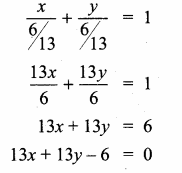Question 10.
A person standing at a junction (crossing) of two straight paths represented by the equations 2x – 3y + 4 = 0 and 3x + 4y – 5 = 0 seek to reach the path whose equation is 6x – 7y + 8 = 0 in the least time. Find the equation of the path that he should follow.
Two straight path will intersect at one point.
Solving this equations

2x – 3y + 4 = 0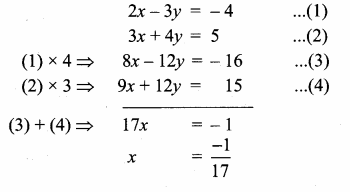Substitute the value of x = [Math Processing Error] in (2)

The point of intersection is (-[Math Processing Error],[Math Processing Error])
Any equation perpendicular to 6x – 7y + 8 = 0 is 7x + 6y + k = 0
It passes through (-[Math Processing Error],[Math Processing Error])
7(-[Math Processing Error]) + 6 ([Math Processing Error]) + k = 0
Multiply by 17
-7 + 6 (22) + 17k = 0
-7 + 132 + 17k = 0
17k = -125 ⇒ k = – [Math Processing Error]
The equation of a line is 7x + 6y – [Math Processing Error] = 0
119x + 102y – 125 = 0
∴ Equation of the path is 119x + 102y – 125 = 0

Tamilnadu, Samacheer, Kalvi, 10th, sslc, Maths, Solutions, Chapter 5, Coordinate Geometry, Ex 5.3,

Question 1.
Find the equation of a straight line passing through the mid-point of a line segment joining the points (1, -5), (4, 2) and parallel to (i) X axis (ii) Y axis
Solution:
Mid point of the line joining to points (1, -5), (4, 2)
Mid point of the line = (\frac{x_{1}+x_{2}}{2}, \frac{y_{1}+y_{2}}{2})
= (\frac { 1+4 }{ 2 } ,\frac { -5+2 }{ 2 } ) = (\frac { 5 }{ 2 } ,\frac { -3 }{ 2 } )

(i) Any line parallel to X-axis. Slope of a line is 0.
Equation of a line is y – y1 = m (x – x1)
y + 32 = 0 (x – 52)
y + 32 = 0 ⇒ 2y+32 = 0
2y + 3 = 0

(ii) Equation of a line parallel to Y-axis is
x = 52 ⇒ 2x = 5
2x – 5 = 0

Question 2.
The equation of a straight line is 2(x – y) + 5 = 0. Find its slope, inclination and intercept on the Y axis.
Solution:
Equation of a line is
2 (x – y) + 5 = 0
2 x – 2y + 5 = 0
-2y = -2x – 5
2y = 2x + 5
y = 2×2 + 52
y = x + 52
Slope of line = 1
Y intercept = 52
tan θ = 1
tan θ = tan 45°
∴ angle of inclination = 45°

Question 3.
Find the equation of a line whose inclination is 30° and making an intercept -3 on the Y axis.
Solution:
Angle of inclination = 30°
Slope of a line = tan 30°
(m) = 13√
y intercept (c) = -3
Equation of a line is y = mx + c
y = 13√ x – 3
3–√ y = x – 3 3–√
∴ x – 3–√ y – 3 3–√ = 0

Question 4.
Find the slope and y intercept of 3x−−√ + (1 – 3–√)y = 3.
Solution:
The equation of a line is 3–√x + (1 – 3–√)y = 3
(1 – 3–√)y = 3–√ x + 3

Question 5.
Find the value of ‘a’, if the line through (-2,3) and (8,5) is perpendicular to y = ax + 2
Solution:
Given points are (-2, 3) and (8, 5)
Slope of a line = y2−y1x2−x1
= 5−38+2 = 210 = 15
Slope of a line y = ax + 2 is “a”
Since two lines are ⊥r
m1 × m2 = -1
15 × a = -1 ⇒ a5 = -1 ⇒ a = -5
∴ The value of a = -5

Question 6.
The hill in the form of a right triangle has its foot at (19,3). The inclination of the hill to the ground is 45°. Find the equation of the hill joining the foot and top.
Solution:
Slope of AB (m) = tan 45°
= 1
Equation of the hill joining the foot and the top is
y – y1 = m(x – x1)
y – 3 = 1 (x – 19)

y – 3 = x – 19
– x + y – 3 + 19 = 0
– x + y + 16 = 0
x – y – 16 = 0
The required equation is x – y – 16 = 0

www.asterclasses.com

Question 7.
Find the equation of a line through the given pair of points.
(i) (2,23) and (−12,-2)
(ii) (2,3) and (-7,-1)
Solution:
(i) Equation of the line passing through the point (2,23) and (−12,-2)

-5(3y – 2) = -8 × 2 (x – 2)
-15y + 10= -16 (x – 2)
-15y + 10= -16x + 32
16x – 15y + 10 – 32 = 0
16x – 15y – 22 = 0
The required equation is 16x – 15y – 22 = 0

(ii) Equation of the line joining the point (2, 3) and (-7, -1) is

-9 (y – 3) = -4 (x – 2)
-9y + 27 = – 4x + 8
4x – 9y + 27 – 8 = 0
4x – 9y + 19 = 0
The required equation is 4x – 9y + 19 = 0

Question 8.
A cat is located at the point(-6, -4) in xy plane. A bottle of milk is kept at (5,11). The cat wish to consume the milk travelling through shortest possible distance. Find the equation of the path it needs to take its milk.
Solution:

Equation of the line joining the point is
y−y1y2−y1=x−x1x2−x1
y+411+4 = x+65+6
y+415 = x+611
15(x + 6) = 11(y + 4)
15x + 90 = 11y + 44
15x – 11y + 90 – 44 = 0
15x – 11y + 46 = 0
The equation of the path is 15x – 11y + 46 = 0

Question 9.
Find the equation of the median and altitude of AABC through A where the vertices are A(6,2), B(-5, -1) and C(1,9).
Solution:
(i) To find median

Equation of the median AD is

2(x – 6) = -8 (y – 2)
2x – 12= -8y + 16
2x + 8y – 28 = 0
(÷ by 2) x + 4y – 14 = 0
∴ Equation of the median is x + 4y – 14 = 0
Equation of the altitude is 3x + 5y – 28 = 0

(ii) To find the equation of the altitude

Slope of BC = y2−y1x2−x1
= 9+11+5
= 106 = 53
Slope of the altitude = – 35
Equation of the altitude AD is
y – y1 = m (x – x1)
y – 2 = – 35 (x – 6)
-3 (x – 6) = 5 (y – 2)
-3x + 18 = 5y – 10
-3x – 5y + 18 + 10 = 0
-3x – 5y + 28 = 0
3x + 5y – 28 = 0

www.asterclasses.com

Question 10.
Find the equation of a straight line which has slope −54 and passing through the point (-1,2).
Solution:
Slope of a line (m) = −54
The given point (x1, y1) = (-1, 2)
Equation of a line is y – y1 = m (x – x1)
y – 2= −54 (x + 1)
5(x + 1) = -4(y – 2)
5x +5 = -4y + 8
5x + 4y + 5 – 8 = 0
5x + 4y – 3 = 0
The equation of a line is 5x + 4y – 3 = 0

Question 11.
You are downloading a song. The percenty (in decimal form) of mega bytes remaining to get downloaded in x seconds is given by y = -0.1x + 1.
(i) graph the equation.
(ii) find the total MB of the song.
(iii) after how many seconds will 75% of the song gets downloaded?
Solution:
(i) y = – 0. 1x + 1

(ii) y = -0.1x + 1
x → seconds
y → Mega byte of the song.
The total mega byte of the song is at the beginning that is when x = 0
y = 1 mega byte

In other words y = 25%
y = 0.25
By using the equation we get
0.25 = -0.1x + 1
0.1 x = 0.75
x = 0.750.1 = 7.5 seconds

i.e y = 0
0 = -0.1 x + 1
0.1 x = 1 second
x = 10.1 = 11 × 10
x = 10 seconds

www.asterclasses.com

Question 12.
Find the equation of a line whose intercepts on the x and y axes are given below.
(i) 4, -6
(ii) -5, – 4
Solution:
(i) x intercept (a) = 4; y intercept (b) = – 6
Equation of a line is xa + yb = 1
x4 + y−6 = 1 ⇒ x4 – y6 = 1
(LCM of 4 and 6 is 12)
3x – 2y = 12
3x – 2y – 12 = 0
The equation of a line is 3x – 2y – 12 = 0

(ii) x intercept (a) = -5; y intercept (b) = 34
Equation of a line is xa + yb = 1 ⇒ x−5+y34=1
x−5 + 4y3 = 1
(LCM of 5 and 3 is 15)
– 3x + 20y = 15
– 3x + 20y – 15 = 0
3x – 20y + 15 = 0
∴ Equation of a line is 3x – 20y + 15 = 0

www.asterclasses.com

Question 13.
Find the intercepts made by the following lines on the coordinate axes.
(i) 3x – 2y – 6 = 0
(ii) 4x + 3y + 12 = 0
Solution:
(i) 3x – 2y – 6 =0.
x intercept when y = 0
⇒ 3x – 6 = 0
⇒ x = 2
y intercept when x = 0
⇒ 0 – 2y – 6 = 0
⇒ y = -3
(ii) 4x + 3y + 12 = 0. x intercept when y = 0
⇒ 4x + 0 + 12 = 0
⇒ x = -3
y intercept when x = 0
⇒ 0 + 3y + 12 = 0
⇒ y = -4

www.asterclasses.com

Question 14.
Find the equation of a straight line.
(i) passing through (1,-4) and has intercepts which are in the ratio 2 : 5
(ii) passing through (-8, 4) and making equal intercepts on the coordinate axes.
Solution:
(i) Let the x-intercept be 2a and the y intercept 5 a
The equation of a line is
xa + ya = 1 ⇒ x2a + y5a = 1
The line passes through the point (1, -4)
12a + (−4)5a = 1 ⇒ 12a – 45a = 1
Multiply by 10a
(L.C.M of 2a and 5a is 10a)
5 – 8 = 10a ⇒ -3 = 10a
a = −310
The equation of the line is

Multiply by 3
-5x – 2y = 3 ⇒ -5x – 2y – 3 = 0
5x + 2y + 3 = 0
The equation of a line is 5x + 2y + 3 = 0

(ii) Let the x-intercept andy intercept “a”
The equation of a line is
xa + ya = 1
The line passes through the point (-8, 4)
−8a + 4a = 1
−8+4a = 1
-4 = a
The equation of a line is
x−4 + y−4 = 1
Multiply by -4
x + y = -4
x + y + 4 = 0
The equation of the line is x + y + 4 = 0

Question 1.
What is the slope of a line whose inclination with positive direction of x -axis is
(i) 90°
(ii) 0°
Solution:
Here θ = 90°
Slope (m) = tan θ
Slope = tan 90°
= undefined.

(ii) Here θ = 0°
Slope (m) = tan θ
Slope = tan 0°
= 0

Question 2.
What is the inclination of a line whose slope is
(i) 0
(ii) 1
Solution:
(i) m = 0
tan θ = 0 ⇒ θ = 0°
(ii) m = 1 ⇒ tan θ = tan 45° ⇒ 0 = 45°

Question 3.
Find the slope of a line joining the points
(i) (5,5–√) with the origin
(ii) (sin θ, -cos θ) and (-sin θ, cos θ)
Solution:
(i) The given points is (5,5–√) and (0, 0)
Slope of a line = y2−y1x2−x1 = 0−5√0−5
= 5√5=15√

(ii) The given points is (sin θ, -cos θ) and (-sin θ, cos θ)
Slope of a line = y2−y1x2−x1=cosθ+cosθ−sinθ−sinθ
= 2cosθ−2sinθ = – cot θ

Question 4.
What is the slope of a line perpendicular to the line joining A(5,1) and P where P is the mid-point of the segment joining (4,2) and (-6,4).
Solution:
Mid point of XY = (x1+x22,y1+y22) = (4−62,2+42)
= (−22,62) = (-1, 3)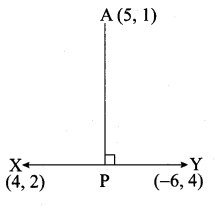Slope of a line = y2−y1x2−x1 = (3−1−1−5)
= 2−6 = – 13

www.asterclasses.com

Question 5.
Show that the given points are collinear: (-3, -4), (7,2) and (12, 5)
Solution:
The vertices are A(-3, -4), B(7, 2) and C(12, 5)
Slope of a line = y2−y1x2−x1
Slope of AB = 2+47+3 = 610 = 35
Slope of BC = 5−212−7 = 35
Slope of AB = Slope of BC = 35
∴ The three points A,B,C are collinear.

Question 6.
If the three points (3, -1), (a, 3) and (1, -3) are collinear, find the value of a.
Solution:
The vertices are A(3, -1), B(a, 3) and C(1, -3)
Slope of a line = y2−y1x2−x1
Slope of AB = 3+1a−3 = 4a−3
Slope of BC = 3+3a−1 = 6a−1
Since the three points are collinear.
Slope of AB = Slope BC
4a−3 = 6a−1
6 (a – 3) = 4 (a – 1)
6a – 18 = 4a – 4
6a – 4a = -4 + 18
2a = 14 ⇒ a = 142 = 7
The value of a = 7

www.asterclasses.com

Question 7.
The line through the points (-2, a) and (9,3) has slope –12 Find the value of a.
Solution:
The given points are (-2, a) and (9, 3)
Slope of a line = y2−y1x2−x1
– 12 = 3−a9+2 ⇒ – 12 = 3−a11
2(3 – a) = -11 ⇒ 6 – 2a = -11
-2a = -11 – 6 ⇒ -2a = -17 ⇒ a = – 172
∴ The value of a = 172

Question 8.
The line through the points (-2, 6) and (4, 8) is perpendicular to the line through the points (8,12) and (x, 24). Find the value of x.
Solution:
Find the slope of the line joining the point (-2, 6) and (4, 8)
Slope of line (m1) = y2−y1x2−x1
= 8−64+2 = 26 = 13
Find the slope of the line joining the points (8, 12) and (x, 24)
Slope of a line (m2) = 24−12x−8 = 12x−8
Since the two lines are perpendicular.
m1 × m2 = -1
13 × 12x−8 = -1 ⇒ 123(x−8)=−1
-1 × 3 (x – 8) = 12
-3x + 24 = 12 ⇒ – 3x = 12 -24
-3x = -12 ⇒ x = 123 = 4
∴ The value of x = 4

www.asterclasses.com

Question 9.
Show that the given points form a right angled triangle and check whether they satisfies Pythagoras theorem.
(i) A(1, -4) , B(2, -3) and C(4, -7)
(ii) L(0, 5), M(9,12) and N(3,14)
Solution:
(i) The vertices are A(1, -4), B(2, -3) and C(4, -7)
Slope of a line = y2−y1x2−x1
Slope of AB = −3+42−1 = 11 = 1
Slope of BC = −7+34−2 = −42 = -2
Slope of AC = −7+44−1 = – 33 = -1
Slope of AB × Slope of AC = 1 × -1 = -1

∴ AB is ⊥r to AC
∠A = 90°
∴ ABC is a right angle triangle
Verification:

20 = 2 + 18
20 = 20 ⇒ Pythagoras theorem verified

(ii) The vertices are L(0, 5), M(9, 12) and N(3, 14)
Slope of a line = y2−y1x2−x1
Slope of LM = 12−59−0 = 79

Slope of MN = 14−123−9 = 2−6 = – 13
Slope of LN = 14−53−0 = 93 = 3
Slope of MN × Slope of LN = – 13 × 3 = -1
∴ MN ⊥ LN
∠N = 90°
∴ LMN is a right angle triangle
Verification:

130 = 90 + 40
130 = 130 ⇒ Pythagoras theorem is verified

Question 10.
Show that the given points form a parallelogram:
A (2.5,3.5), B(10, -4), C(2.5, -2.5) and D(-5, 5).
Solution:
Let A(2.5, 3.5), B(10, -4), C(2.5, -2.5) and D(-5, 5) are the vertices of a parallelogram.

Slope of AB = Slope of CD = -1
∴ AB is Parallel to CD ……(1)

Slope of BC = Slope of AD
∴ BC is parallel to AD
From (1) and (2) we get ABCD is a parallelogram.

www.asterclasses.com

Question 11.
If the points A(2, 2), B(-2, -3), C(1, -3) and D(x, y) form a parallelogram then find the value of x and y.
Solution:
Let A(2, 2), B(-2, -3), C(1, -3) and D(x, y) are the vertices of a parallelogram.

Slope of a line = y2−y1x2−x1
Slope of AB = −3−2−2−2 = −5−4 = 54
Slope of BC = −3+3−2−1 = 0−3 = 0
Slope of CD = y+3x−1
Since ABCD is a parallelogram
Slope of AB = Slope of CD
54 = y+3x−1
5(x – 1) = 4 (y + 3)
5x – 5 = 4y + 12
5x – 4y = 12 + 5
5x – 4y = 17 ……(1)
Slope of BC = Slope of AD
0 = y−2x−2
y – 2 = 0
y = 2
Substitute the value of y = 2 in (1)
5x – 4(2) = 17
5x -8 = 17 ⇒ 5x = 17 + 18
5x = 25 ⇒ x = 255 = 5
The value of x = 5 and y = 2.

Question 12.
Let A(3, -4), B(9, -4) , C(5, -7) and D(7, -7). Show that ABCD is a trapezium.
Solution:
Let A(3, -4), B(9, -4), C(5, -7) and D(7, -7) are the vertices of a quadrilateral.

Slope of a line = y2−y1x2−x1
Slope of AB = −4+49−3 = 06 = 0
Slope of BC = −7+45−9 = −3−4 = 34
Slope of CD = −7+77−5 = 02 = 0
Slope of AD = −7+47−3 = −34 = – 34
The slope of AB and CD are equal.
∴ AB is parallel to CD. Similarly the slope of AD and BC are not equal.
∴ AD and BC are not parallel.
∴ The Quadrilateral ABCD is a trapezium.

Question 13.
A quadrilateral has vertices at A(-4, -2), B(5, -1) , C(6, 5) and D(-7, 6). Show that the mid-points of its sides form a parallelogram.
Solution:
Let A(-4, -2), B(5, -1), C(6, 5) and D(-7, 6) are the vertices of a quadrilateral.

Slope of EF = Slope of GH = 710
∴ EF || GH …….(1)
Slope of FG= Slope of EH = – 712
∴ FG || EH ……(2)
From (1) and (2) we get EFGH is a parallelogram.
The mid point of the sides of the Quadrilateral ABCD is a Parallelogram.

www.asterclasses.com

Question 14.
PQRS is a rhombus. Its diagonals PR and QS intersect at the point M and satisfy QS = 2PR. If the coordinates of S and M are (1, 1) and (2, -1) respectively, find the coordinates of P.
Solution:
Slope of a line = y2−y1x2−x1
Slope of SM = 1+11−2 = 2−1 = -2
Slope of PM = 12 (Since SM and PM are ⊥r)
Let the point p be (a,b)
Slope of PM = 12
b+1a−2 = 12 ⇒ a – 2 = 2b + 2

a – 2b = 4
a = 4 + 2b ……(1)
Given QS = 2PR
QS2 = PR
∴ SM = PR
SM = 2PM (PR = 2PM)

Squaring on both sides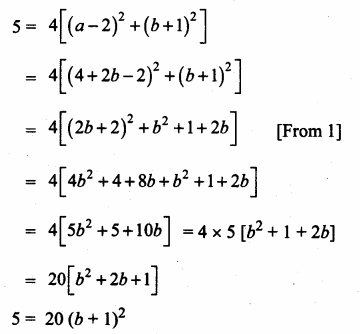∴ (b + 1)2 = 14 ⇒ b + 1 = ± 12
b = 12 – 1 (or) b = – 12 – 1
= – 12 – 1 (or) b = –12 – 1
= – 12 (or) – 32
a = 4 + 2b
a = 4 + 2 (−12)
a = 3
a = 4 + 2 (−32)
a = 4 – 3
a = 1
The point of p is (3,−12) (or) (1,−32)

Tamilnadu, Samacheer, Kalvi, 10th, sslc, Maths, Solutions, Chapter 5, Coordinate Geometry, Ex 5.1,

Question 1.
Find the area of the triangle formed by the points
(i) (1,-1), (-4, 6) and (-3, -5)
(ii) (-10, -4), (-8, -1) and (-3, -5)
Solution:
(i) Let the vertices A (1, -1), B (-4, 6) and C (-3, -5)

= 12 [(6 + 20 + 3) – (4 – 18 – 5)] = 12 [29 – (-19)] = 12 [29 + 19]
= 12 × 48 = 24 sq. units.
Area of ∆ABC = 24 sq. units

(ii) Let the vertices be A(-10, -4), B(-8 -1) and C(-3, -5)

Area of ∆ABC = 12[(x1y2 + x2y3 + x3y1) – (x2y1 + x3y2 + x1y3)]
= 12 [(50 + 3 + 32) – (12 + 40 + 10)]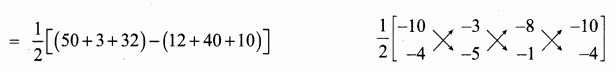= 12 [85 – (62)] = 12  = 11.5
Area of ∆ACB = 11.5 sq.units

www.asterclasses.com

Question 2.
Determine whether the sets of points are collinear?
(i) (-12,3)
(ii) (a,b + c), (b,c + a) and (c,a + b)
Solution:
(i) Let the points be A (-12,3), B (-5, 6) and C(-8, 8)
Area of ∆ABC = 12 [(x1y2 + x2y3 + x3y1) – (x2y1 + x3y3 + x1y3)]
= 12 [(- 3 – 40 – 24) – (-15 – 48 – 4)]

= 12 [-67 + 67] = 12 × 0 = 0
Area of a ∆ is 0.
∴ The three points are collinear.

(ii) Let the points be A (a, b + c), B (b, c + a) and C (c, a + b)
Area of the triangle = 12 [(x1y2 + x2y3 + x3y1) – (x2y1 + x3y2 + x1y3)]

Since the area of a triangle is 0.
∴ The given points are collinear.

www.asterclasses.com

Question 3.
Vertices of given triangles are taken in order and their areas are provided aside. In each case, find the value of ‘p’

Solution:
(i) Let the vertices be A (0,0) B (p, 8), c (6, 2)
Area of a triangle = 20 sq. units
12 [(x1y2 + x2y3 + x3y1) – (x2y1 + x3y2 + x1y3)] = 20

12 [(0 + 2p + 0) – (0 + 48 + 0)] = 20
12 [2p – 48] = 20
2p – 48 = 40 ⇒ 2p = 40 + 48
p = 882 = 44
The value of p = 44

(ii) Let the vertices be A (p, p), B (5, 6) and C (5, -2)
Area of a triangle = 32 sq. units
12 [(x1y2 + x2y3 + x3y1) – (x2y1 + x3y2 + x1y3)] = 32

www.asterclasses.com

Question 4.
In each of the following, find the value of ‘a’ for which the given points are collinear.
(i) (2,3), (4, a) and (6, -3)
(ii) (a, 2 – 2a), (-a + 1, 2a) and (-4 -a, 6 – 2a).
Solution:
(i) Let the points be A (2, 3), B(4, a) and C(6, -3).
Since the given points are collinear.
Area of a triangle = 0
12 [(x1y2 + x2y3 + x3y1) – (x2y1 + x3y2 + x1y3)] = 0)

(ii) Let the points be A (a, 2 – 2a), B (-a + 1, 2a) C (-4 -a, 6 – 2a).
Since the given points are collinear.
Area of a ∆ = 0

6a2 – 2a – 2 – (-2a2 – 6a + 2) = 0
6a2 – 2a – 2 + 2a2 + 6a – 2 = 0
8a2 + 4a – 4 = 0 (Divided by 4)
2a2 + a – 1 = 0
2a2 + 2a – a – 1 = 0
2a (a + 1) – 1 (a + 1) = 0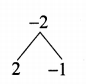(a + 1) (2a – 1) = 0
a + 1 = 0 (or) 2a – 1 = 0
a = -1 (or) 2a = 1 ⇒ a = 12
The value of a = -1 (or) 12

www.asterclasses.com

Question 5.
Find the area of the quadrilateral whose vertices are at
(i) (-9, -2), (-8, -4), (2, 2) and (1, -3)
(ii) (-9, 0), (-8,6), (-1, -2) and (-6, -3)
Solution:
(i) Let the vertices A (-9, -2), B(-8, -4), C(2, 2) and D(1, -3).
Plot the vertices in a graph.

[Note: Consider the points in counter clock wise order]

Area of the Quadrilateral ABDC = 12 [36 + 24 + 2 – 4 – (16 – 4 – 6 – 18)]
= 12 [58 – (-12)] – 12[58 + 12]
= 12 × 70 = 35 sq. units 2
Area of the Quadrilateral = 35 sq. units

www.asterclasses.com

(ii) Let the vertices A(-9, 0), B(-8, 6), C(-1, -2) and D(-6, -3)
Plot the vertices in a graph and take them in counter – clock wise order.

= 12 [33 + 35] = 12 × 68 = 34 sq. units
Area of the Quadrilateral = 34 sq. units

www.asterclasses.com

Question 6.
Find the value of k, if the area of a quadrilateral is 28 sq. units, whose vertices are (-4, -2), (-3, k), (3, -2) and (2, 3)
Solution:
Let the vertices A (-A, -2), B (-3, k), C (3, -2) and D (2, 3)
Area of the Quadrilateral = 28 sq. units
12 [(x1y2 + x2y3 + x3y4 + x4y1) – (x2y1 + x3y2+ x4y3 + x1y4)] = 28

-7k + 21 = 56
-7k = 56 – 21
-7k = 35 ⇒ 7k = – 35
k = – 357 = -5
The value of k = -5

www.asterclasses.com

Question 7.
If the points A(-3, 9), B(a, b) and C(4, -5) are collinear and if a + b = 1, then find a and b.
Solution:
Since the three points are collinear
Area of a ∆ = 0

-3b – 5a + 36 – 9a – 4b – 15 = 0
-7b – 14a + 21 = 0
(÷ by 7) – b – 2a + 3 = 0
2a + b – 3 = 0Substitute the value of a = 2 in (2) ⇒ 2 + b = 1
b = 1 – 2 = -1
The value of a = 2 and b = -1

Question 8.
Let P(11, 7), Q(13.5, 4) and R(9.5, 4) be the midpoints of the sides AB, BC and AC respectively of ∆ABC . Find the coordinates of the vertices A, B and C. Hence find the area of ∆ABC and compare this with area of ∆PQR.
Solution:
Let the vertices of the ∆ABC be A(x1,y1), B(x2,y2), C(x3,y3)

www.asterclasses.com

Question 9.
In the figure, the quadrilateral swimming pool shown is surrounded by concrete patio. Find the area of the patio.Solution: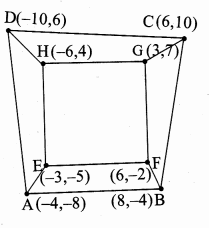= 12 [(x1y2 + x2y3 + x3y4 + x4y1) – (x2y1 + x3y2 + x4y3 + x1y4)]
= 12 [(16 + 80 + 36 + 80) – (-64 – 24 – 100 – 24)]
= 12 [212 – (-212)]

= 12 [212 + 212] = 12  = 212 sq. units= 12 [90 – (-90)]

= 12 [90 + 90]
= 12 × 180 = 90 sq. units
Area of the patio = Area of the Quadrilateral ABCD – Area of the Quadrilateral EFGH
= (212 – 90) sq. units
Area of the patio = 122 sq. units

www.asterclasses.com

Question 10.
A triangular shaped glass with vertices at A(-5, -4), B(l, 6) and C(7, -4) has to be painted. If one bucket of paint covers 6 square feet, how many buckets of paint will be required to paint the whole glass, if only one coat of paint is applied.
Solution:
Given the vertices of the triangular glass is A (-5, -4), B (1, 6), and C (7, -4)

= 12 [(20 + 42 – 4) – (-28 – 4 – 30)]
= 12 [58 – (-62)]
= 12 [58 + 62]
= 12 × 120 = 60 sq. feet
Number of cans to paint 6 square feet = 1
∴ Number of cans = 606 = 10 ⇒ Number of cans = 10

www.asterclasses.com

Question 11.
In the figure, find the area of
(i) triangle AGF
(ii) triangle FED

Solution:
Area of a triangle = 12 [(x1y2 + x2y3 + x3y1) – (x2y1 + x3y2 + x1y3)]
(i) Area of ∆AGF = 12 [(-2.5 – 13.5 – 6) – (-13.5 – 1 – 15)]
= 12 [-22 – (-29.5)]

= 12 [-22 + 29.5]
= 12 × 7.5 = 3.75 sq.units

(ii) Area of ∆FED = 12 [(-2 + 4.5 + 3) – (4.5 + 1 – 6)]
= 12 [5.5 – (-0.5)]

= 12 [5.5 + 0.5] = 12 × 6 = 3 sq.units

(iii)

= 12 [(4 + 2 + 0.75 + 9) – (-4 -1.5 – 4.5 -2)]
= 12 [15.75 + 12]
= 12 [27.75] = 13.875
= 13.88 sq. units

Tamilnadu, Samacheer, Kalvi, 10th, sslc, Maths, Solutions, Chapter 4, Geometry, Additional, Questions,

Question 1.
If a straight line intersects the sides AB and AC of a ∆ABC at D and E respectively and is parallel to BC, then AEAC = …………
(3) DEBC

Hint:
By BPT theorem,

Question 2.
In ∆ABC, DE is || to BC, meeting AB and AC at D and E. If AD = 3 cm, DB = 2 cm and AE = 2.7 cm, then AC is equal to ……..
(1) 6.5 cm
(2) 4.5 cm
(3) 3.5 cm
(4) 3.5 cm
(2) 4.5 cm
Hint:
By BPT theorem,

32 = 2.7EC
∴ EC = 2.7×23 = 1.8 CM
AC = AE + EC
= 2.7 + 1.8 = 4.5 cm

Question 3.
In ∆PQR, RS is the bisector of ∠R. If PQ = 6 cm, QR = 8 cm, RP = 4 cm then PS is equal to …………..
(1) 2 cm
(2) 4 cm
(3) 3 cm
(4) 6 cm
(2) 2 cm
Hint:

By ABT theorem,
PSSQ = PRQR ⇒ x6−x = 48
24 – 4x = 8x ⇒ 24 = 12x
x = 2
PS = 2 cm

Question 4.
In figure, if ABAC = BDDC, ∠B = 40° and ∠C = 60°, then ∠BAD = ……………
(1) 30°
(2) 50°
(3) 80°
(4) 40°
(4) 40°
Hint:
ABAC = BDDC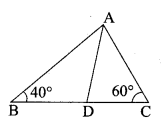AD is the internal bisector of ∠BAC
∠A + ∠B + ∠C = 180°
∠A + 40° + 60° = 180° ⇒ ∠A = 80°
∴ ∠BAC = 802 = 40°

Question 5.
In the figure, the value x is equal to …………
(1) 4.2
(2) 3.2
(3) 0.8
(4) 0.4

(2) 3.2
Hint:
By Thales theorem
(DE || BC)
x8 = 410 ⇒ x = 8×410 = 3.2

www.asterclasses.com

Question 6.
In triangles ABC and DEF, ∠B = ∠E, ∠C = ∠F, then ………….
(1) ABDE = CAEF
(2) BCEF = ABFD
(3) ABDE = BCEF
(4) CAFD = ABEF
(3) ABDE = BCEF
Hint:

Question 7.
From the given figure, identify the wrong statement.

(2) ∆ABD ~ ∆ABC
(3) ∆BDC ~ ∆ABC
(2) ∆ ABD ~ ∆ ABC

www.asterclasses.com

Question 8.
If a vertical stick 12 m long casts a shadow 8 m long on the ground and at the same time a tower casts a shadow 40 m long on the ground, then the height of the tower is …………..
(1) 40 m
(2) 50 m
(3) 75 m
(4) 60 m
(4) 60 m
Hint:

ABDE = BCEF
12h = 840; h = 40×128 = 60

Question 9.
The sides of two similar triangles are in the ratio 2:3, then their areas are in the ratio ………….
(1) 9 : 4
(2) 4 : 9
(3) 2 : 3
(4) 3 : 2
(2) 4 : 9
Hint:
Ratio of the Area of two similar triangle
= 22 : 32 = 4 : 9
[squares of their corresponding sides]

Question 10.
Triangles ABC and DEF are similar. If their areas are 100 cm2 and 49 cm2respectively and BC is 8.2 cm then EF = …………….
(1) 5.47 cm
(2) 5.74 cm
(3) 6.47 cm
(4) 6.74 cm
(2) 5.74 cm
Hint:

Question 11.
The perimeters of two similar triangles are 24 cm and 18 cm respectively.
If one side of the first triangle is 8 cm, then the corresponding side of the other triangle is ………
(1) 4 cm
(2) 3 cm
(3) 9 cm
(4) 6 cm
(4) 6 cm
Hint:

Question 12.
A point P is 26 cm away from the centre O of a circle and PT is the tangent drawn from P to the circle 10 cm, then OT is equal to …………
(1) 36 cm
(2) 20 cm
(3) 18 cm
(4) 24 cm
(4) 24 cm
Hint:

OT2 = OP2 – PT2
= 262 – 102
= (26 + 10) (26 – 10)
OT2 = 36 × 16
OT = 6 × 4 = 24 cm

Question 13.
In the figure, if ∠PAB = 120° then ∠BPT = ……….

(1) 120°
(2) 30°
(3) 40°
(4) 60°
(4) 60°
Hint:
∠BCP + ∠PAB = 180°
(sum of the opposite angles of a cyclic quadrilateral)
∠BCP = 180° – 120° = 60°
∠BPT = 60°
(By tangent chord theorem)

Question 14.
If the tangents PA and PB from an external point P to circle with centre O are inclined to each other at an angle of 40°, then ∠POA = ………………
(1) 70°
(2) 80°
(3) 50°
(4) 60°
(1) 70°
Hint:
∠OPA = 402 = 20°
In ∆OAP.
∠POA + ∠OAP + ∠APO = 180°
(sum of the angles of a triangle)

∠POA + 90° + 20° = 180°
∠POA = 180° – 110° = 70°

Question 15.
In the figure, PA and PB are tangents to the circle drawn from an external point P. Also CD is a tangent to the circle at Q. If PA = 8 cm and CQ = 3 cm, then PC is equal to ……….

(1) 11 cm
(2) 5 cm
(3) 24 cm
(4) 38 cm
(2) 5 cm
Hint:
PA = PB (tangent of a circle)
PB = 8 cm
PC + BC = 8
PA + QC = (BC = QC tangent)
PC + 3 = 8
∴ PC = 8 cm – 3 cm = 5 cm

Question 16.
∆ABC is a right angled triangle where ∠B = 90° and BD ⊥ AC. If BD = 8 cm, AD = 4 cm, then CD is …………
(1) 24 cm
(2) 16 cm
(3) 32 cm
(4) 8 cm
(2) 16 cm
Hint:
∆DCB ~ ∆DBA
DCDB = DBDA

DB2 = DC × DA
82 = DC × 4
DC = 644 = 16 cm

Question 17.
The areas of two similar triangles are 16 cm2 and 36 cm2 respectively. If the altitude of the first triangle is 3 cm, then the corresponding altitude of the other triangle is
(1) 6.5 cm
(2) 6 cm
(3) 4 cm
(4) 4.5 cm
(4) 4.5 cm
Hint:

Question 18.
The perimeter of two similar triangles ∆ABC and ∆DEF are 36 cm and 24 cm respectively. If DE =10 cm, then AB is …………
(1) 12 cm
(2) 20 cm
(3) 15 cm
(4) 18 cm
(3) 15 cm
Hint:
Perimeter of

Question 19.
In the given diagram θ is ………….
(1) 15°
(2) 30°
(3) 45°
(4) 60°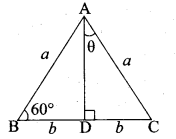(2) 30°
Hint:
∠BAD = 180° – 150° = 30°
= 180° – 150° = 30°
∠DAC = θ = 30°

Question 20.
If AD is the bisector of ∠A then AC is …………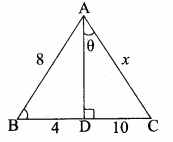(1) 12
(2) 16
(3) 18
(4) 20
(4) 20
Hint. In ∆ABC, AD is the internal bisector of ∠A
BDDC = ABAC
410 = 8x
4x = 10 × 8
x = 10×84 = 20 cm

Question 21.
In ∆ABC and ∆DEF, ∠A = ∠E and ∠B = ∠F. Then AB : AC is ………….
(1) DE : DF
(2) DE : EF
(3) EF : ED
(4) DF : EF
(3) EF : ED
Hint:

ABEF = BCFD = ACED

Question 22.
Two circles of radius 8.2 cm and 3.6 cm touch each other externally, the distance between their centres is ………….
(1) 1.8 cm
(2) 4.1 cm
(3) 4.6 cm
(4) 11.8 cm
(4) 11.8 cm
Hint:
Distance between the two centres

= r1 + r2
= 8.2 + 3.6
= 11.8 cm

Question 23.
In the given diagram PA and PB are tangents drawn from P to a circle with centre O. ∠OPA = 35° then a and b is …………

(1) a = 30°, b = 60°
(2) a = 35°, b = 55°
(3) a = 40°, b = 50°
(4) a = 45°, b = 45°
(2) a = 35°, b = 55°
Hint:
∠OAP = 90° (tangent of the circle)
∠AOP + ∠OPA + ∠PAO = 180°
b + 35° + 90° = 180°
b = 180° – 125°
= 55°
OP is the angle bisector of ∠P
∴ a = 35°

### II. Answer the following questions

Question 1.
The image of a man of height 1.8 m, is of length 1.5 cm on the film of a camera. If the film is 3 cm from the lens of the camera, how far is the man from the camera?
Solution:
Let AB be the height of the man, CD be the height of the image of the man of height 1.8 m (180 cm). LM be the distance between man and lens. LN be the distance between Lens and the film.

Given, AB = 1.8 m (180 cm)
CD = 1.5 cm and LN = 3 cm
Consider ∆ LAB and ∆ LCD
∠ALB = ∠DLC (vertically opposite angles)
∠LAB = ∠LDC (alternate angles) (AB || CD)
∴ ∆ LAB ~ ∆ LDC (AA similarity)
∴ ABCD = LMLN ⇒ 1801.5 = x3 ⇒ 1.5x = 180 × 3
x = 180×31.5 = 180×3×1015
x = 360 cm (or) 3.6 m
∴ The distance between the man and the camera = 3.6 cm

Question 2.
A girl of height 120 cm is walking away from the base of a lamp-post at a speed of 0. 6 m/sec. If the lamp is 3.6 m above the ground level, then find the length of her shadow after 4 seconds.
Solution:
Let AB be the height of the lamp-post above the ground level.
AB = 3.6 m = 360 cm
Let CD be the height of the girl.
CD = 1.2 m = 120 cm
The distance travelled by the girl in 4 seconds (AC)
= 4 × 0.6 = 2.4m = 240 cm
Consider ∆ECD and ∆EAB
Given (CD || AB)
∠EAB = ∠ECD = 90°
∠E is common

∴ ∆ EAB = ∆ ECD = 90°
ABCD = AECE
= x+240x ⇒ 3 = x+240x
3x = x + 240
2x = 240 ⇒ x = 2402 = 120 cm
∴ Lenght of girls shadow after 4 seconds = 120 cm

Question 3.
In ∆ ABC, AB = AC and BC = 6 cm. D is a point on the side AC such that AD = 5 cm and CD = 4 cm. Show that ∆BCD ~ ∆ACB and hence find BD.
Solution:
Given, In ∆ ABC, AB = AC = 9 cm and BC = 6 cm, AD = 5 cm and CD = 4 cm
BCAC = 69 = 23 …..(1)
CDCB = 46 = 23 ……..(2)

From (1) and (2) we get,
BCAC = CDCB
In ∆ BCD and ∆ ACB
∠C = ∠C (common angle)
BCAC = CDCB
∴ ∆ BCD ~ ∆ ACB
BDAB = BCAC ⇒ BD9 = 69 ⇒ ∴ BD = 6 cm.

Question 4.
The lengths of three sides of a triangle ABC are 6 cm, 4 cm and 9 cm. ∆PQR ~ ∆ABC. One of the lengths of sides of APQR is 35 cm. What is the greatest perimeter possible for ∆PQR?
Solution:
Given, ∆ PQR ~ ∆ ABC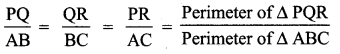Perimeter of ∆ ABC = 6 + 4 + 9 = 19 cm
When the perimeter of ∆ PQR is the greatest only the corresponding side QR must be equal to 35 cm

Question 5.
A man sees the top of a tower in a mirror which is at a distance of 87.6 m from the tower. The mirror is on the ground, facing upward. The man is 0.4 m away from the mirror, and the distance of his eye level from the ground is 1.5 m. How tall is the tower? (The foot of man, the mirror and the foot of the tower lie along a straight line).
Solution:
Let AB and ED be the heights of the man and the tower respectively. Let C be the point of incidence of the tower in the mirror.
In ∆ ABC and ∆ EDC, we have

www.asterclasses.com

Question 6.
In ∆ PQR, given that S is a point on PQ such that ST || QR and PSSQ = 35. If PR = 5.6 cm, then find PT.
Solution:
In ∆ PQR, we have ST || QR and by Thales theorem,
PSSQ = PTTR …….(1)
Let PT = x
Thus, TR = PR – PT = 5.6 – x

From (1), we get PT = TR (PSSQ)
x = (5.6 – x) (35)
5x = 16.8 – 3x
8x = 16.8
x = 16.88 = 2.1
That is PT = 2.1 cm

Question 8.
In the figure AC || BD and CE || DF. if OA = 12 cm, AB = 9cm, OC = 8 cm and EF = 4.5 cm, then find FO.
Solution: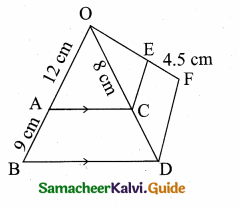In OBD, AC || BD
∴ OAAB = OCCD (By Thales theorem)
129 = 8CD
∴ CD = 9×812 = 6 CM
In ODF, CE || DF
OCCD = OEEF (By Thales theorem)
86 = OE4.5 ⇒ OE = =8×4.56 = 6 cm
FO = FE + EO = 4.5 + 6 = 10.5 cm
∴ The value of FO = 10.5 cm

Question 9.
Check whether AD is the bisector of ∠A of ∆ABC in each of the following.
(i) AB = 4 cm, AC = 6 cm, BD 1.6 cm, and CD = 2.4 cm.
Solution:
BDDC = 1.62.4 = 1624 = 23 …..(1)
ABAC = 46 = 23 ……..(2)
From (1) and (2) we get,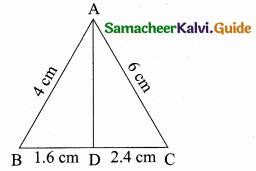BDDC = ABAC
By the converse of angle bisector theorem we have,
∴ AD is the internal bisector of ∠A

(ii) AB = 6 cm, AC = 8 cm, BD = 1.5 cm and CD = 3 cm
Solution:
BDDC = 1.53 = 0.5 …….(1)
ABAC = 68 = 34 …….(2)From (1) and (2) we get,
BDDC ≠ ABAC
Hence AD is not the bisector of ∠A.

Question 10.
In a ∆ABC, AD is the internal bisector of ∠A, meeting BC at D. If AB 5.6 cm, AC = 6 cm and DC = 3 cm, find BC.
Solution:
Given, AB = 5.6 cm, AC = 6 cm, DC = 3 cm
Let BD be x
In ∆ ABC, AD is the internal bisector of ∠A.
By Angle bisector theorem, we have,BDDC = ABAC ⇒ x3 = 5.66
x = 3×5.66 = 2.8 cm
∴ BC = BD + DC = 2.8 + 3 = 5.8 cm
Length of BC = 5.8 cm

Question 11.
In the figure, tangents PA and PB are drawn to a circle with centre O from an external point P. If CD is a tangent to the circle at E and AP = 15 cm, find the perimeter of ∆PCD.
Solution:
We know that the lengths of the two tangents from an exterior point to a circle are equal.
∴ CA = CE, DB = DE and PA = PB
Now, the perimeter of ∆PCD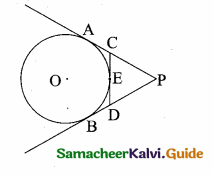= PC + CD + DP
= PC + CE + ED + DP
= PC + CA + DB + DP
= PA + PB = 2 PA (PB = PA)
Thus, the perimeter of APCD = 2 × 15 = 30 cm

Question 12.
ABCD is a quadrilateral such that all of its sides touch a circle. If AB = 6 cm, BC = 6.5 cm and CD = 7 cm, then find the length of AD.
Solution:
Let P, Q, R and S be the points where the circle touches the quadrilateral.
We know that the lengths of the two tangents drawn from an exterior point to a circle are equal. Thus, we have,AP = AS, BP = BQ, CR = CQ and DR = DS
Hence, AP + BP + CR + DR = AS + BQ + CQ + DS
⇒ AB + CD = AD + BC
⇒ AD = AB + CD – BC
= 6 + 7 – 6.5 = 6.5

Question 13.
A man goes 10 m due east and then 24 m due north. Find the distance from the starting point.
Solution:
Let the initial position of the man be “O” and the final position be B.
In the ∆AOB,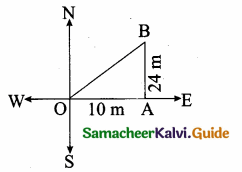OB2 = OA2 + AB2
OB2 = 102 + 242
= 100 + 576 = 676
OB = 676−−−√ = 26 m
The man is at a distance of 26 m from the starting point.

Question 14.
Suppose AB, AC and BC have lengths 13, 16 and 20 respectively. If AFFB = 45 and CEEA= 512 Find BD and DC.
Solution:
Given that AB = 13, AC = 16 and BC = 20. Let BD = JC and DC = y.
Using Ceva’s theorem we haveBDDC × CEEA × AFFB = 1 ………(1)
Substitute the given values
xy × 512 × 45 = 1 ⇒ xy × 13 = 1
xy = 3 ⇒ x = 3y
x – 3y = 0 ……(2)
Given BC = 20
x + y = 20 …..(3)
Subtract (2) and (3)Question 15.
ABC is a right – angled triangle at B. Let D and E be any two points on AB and BC respectively. prove that AE2 + CD2 = AC2 + DE2.
Solution:
In the right ∆ ABE right- angled at B.
AE2 = AB2 + BE2 …..(1)
In the right ∆ DBC,CD2 = BD2 + BC2 ………(2)
Adding (1) and (2) we get
AE2 + CD2 = AB2 + BE2 + BD2 + BC2
= (AB2 + BC2) + (BC2 + BD2)
AE2 + CD2 = AC2 + DE2
Hence it is proved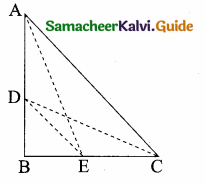[AC2 = AB2 + BC2]
[DE2 = BE2 + BD2]

### III. Answer the following questions

Question 1.
A point O in the interior of a rectangle ABCD is joined to each of the vertices A, B, C and D. Prove that OA2 + OC2 = OB2 + OD2.
Solution:
Given: O is any point inside the rectangle ABCD.
To prove: OA2 + OC2 = OB2 + OD2
Construction: Through “O” draw EF || AB.
Proof: Using Pythagoras theoremIn the right ∆OEA,
∴ OA2= OE2 + AE2 …(1)
(By Pythagoras theorem)
In the right ∆OFC,
OC2 = OF2 + FC2 …(2)
(By Pythagoras theorem)
OB2 = OF2 + FB2 … (3)
(By Pythagoras theorem)
In the right ∆OED,
OD2 = OE2 + ED2 … (4)
(By Pythagoras theorem)
By adding (3) and (4) we get
OB2 + OD2 = OF2 + FB2 + OE2 + ED2
= (OE2 + FB2) + (OF2 + ED2)
= (OE2 + EA2) + (OF2 + FC2)
[FB = EA and ED = FC]
OB2 + OD2 = OA2 + OC2 using (1) and (2)

Question 2.
A lotus is 20 cm above the water surface in a pond and its stem is partly below the water surface. As the wind blew, the stem is pushed aside so that the lotus touched the water 40 cm away from the original position of the stem. How much of the stem was below the water surface originally?
Solution:
Let O be the bottom of the stem immersed in water.
Let B be the lotus, AB be the length of the stem above the water surface
AB = 20 cm
Let OA be the length of the stem below the water surface
Let OA = x cm
Let C be the point where the lotus touches the water surface when the wind blow.
OC = OA + AB
OC = x + 20 cm
In ∆ AOC, OC2 = OA2 + AC2
(x + 20)2 = x2 + 402
x2 + 400 + 40x = x2 + 160040x = 1600 – 400
40x = 1200
x = 120040 = 30 cm
The stem is 30 cm below the water surface.

Question 3.
In the figure, DE || BC and ADBD = 35, calculate the value of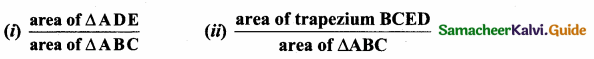Solution:
(i) Given, DE || BC and ADBD = 35
Let AD = 3k and BD = 5k; AB = 3k + 5k = 8k
∠A = ∠A (common angle)
Since DE || BC
∴ ∆ ABC ~ ∆ ADE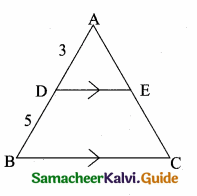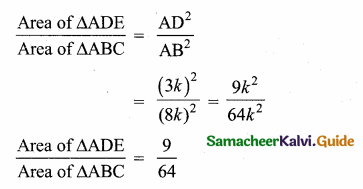(ii) Let Area of ∆ ADE be 9k and Area of ∆ ABC be 64 k
Area of ∆ BCED = Area of ∆ ABC – Area of ∆ ADE
= 64 k – 9 k
= 55 k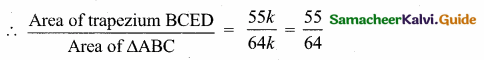Question 4.
A boy is designing a diamond shaped kite, as shown in the figure where AE = 16 cm, EC = 81 cm. He wants to use a straight cross bar BD. How long should it be?
Solution:
Let in AB be “x”, BE be “z” and BC be “y”
In the right ∆ AEB,
AB2 = AE2 + BE2
x2 = 162 + Z2 …….(1)
In the right ∆ BEC,
BC2 = EC2 + BE2
y2 = 812 + z2 ……(2)In the right ∆ ACD,
972 = x2 + y2 ….(3)
Add (1) and (2) ⇒ x2 + y2 = 162 + z2 + 812+ z2
x2 + y2 = 2z2 + 162 + 812
972 = 2z2 + 162 + 812 (from 3)
9409 = 2z2 + 256 + 6561
= 2z2 + 6817
2z2 = 9409 – 6817 = 2592
z2 = 25922 = 1296
z = 1296−−−−√ = 36
∴ Length of cross bar BD = 2 × BE = 2 × 36 = 72 cm

Question 5.
Find the unknown values in each of the following figures. All lengths are given in centimetres (All measures are not in scale)

Solution:
(i) In ∆ ABC and ∆ ADE,
∠A = ∠A (common angle)
∠ABC = ∠ADE (corresponding angle) [BC || DE]
∆ ABC ~ ∆ ADE (By AAA similarity)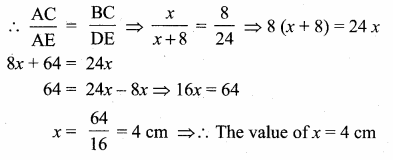In ∆ EAG and ∆ ECF,
∠E || ∠E (common angle)
∠ECF = ∠EAG (corresponding angle)
Given CF || AG
∆ EAG ~ ∆ ECF
ECEA = CFAG ⇒ 8x+8 = 6y ⇒ 812 = 6y (x = 4)
8y = 6 × 12
y = 12×68 = 9 cm
∴ The value of x = 4 cm and y = 9 cm.

(ii) In ∆ HBC and ∆ HFG,
∠H = ∠H (common angle)
∠HFG = ∠HBC (corresponding angle)
Given FG || BC
HFHB = FGBC ⇒ 410 = x9 ⇒ 10x = 36
x = 3.6 cm
In ∆ FBD and ∆ FHD,
∠BFD = ∠HFG (vertically opposite angle)
∠FBD = ∠FHG (Alternate angles)
By AA similarity
∆ FBD ~ ∆ FHG
FGFD = FHFB ⇒ x3+y = 46
4 (3 + y) = 3.6 × 6
3 + y = 3.6×64 = 5.4 ⇒ y = 5.4 – 3 = 2.4 cm
In ∆ AEG and ∆ ABC,
∠A = ∠A (common angle)
∠AEG = ∠ABC (corresponding angles)
Given EG || BC
AEAB = EGBC
zz+5 = x+y9 ⇒ zz+5 = 3.6+2.49
zz+5 = 69 ⇒ zz+5 = 23
3z = 2z + 10 ⇒ 3z – 2z = 10 ⇒ z = 10
∴ The values of x = 3.6 cm, y = 2.4 cm and z = 10 cm.

Question 6.
The internal bisector of ∠A of AABC meets BC at D and the external bisector of ∠A meets BC produced at E. Prove that BDBE = CDCE
Solution:
Given: In ∆ ABC, AD is the internal bisector of ∠A meets BC at D. AE is the external bisector of ∠A meets BC produced to E.
To Proof: BDBE = CDCE

Proof: In ∆ ABC, AD is the internal bisector of ∠A.
By ABT theorem we have,
∴ BECD = ABAC ……….(1)
In ∆ABC, AE is the external bisector of ∠A.
By ABT theorem we have,
∴ BECE = ABAC ……….(2)
From (1) and (2) we get
BDCD = BECE ⇒ ∴ BDBE = CDCE

Question 7.
In a quadrilateral ABCD, the bisectors of ∠B and ∠D intersect on AC at E. Prove that ABBC = ADDC
Solution:
Given: ABCD is a quadrilateral. BE is the bisector of ∠B intersecting AC at E, DE is the bisector of ∠D intersecting AC at E.
Proof: In ∆ABC, BE is the internal bisector of ∠D.
By Angle bisector theorem we have,
AEEC = ABBC ……..(1)

Question 9.
D is the midpoint of the side BC of AABC. If P and Q are points on AB and on AC such that DP bisects ∠BDA and DQ bisects ∠ADC, then prove that PQ || BC.
Solution:
In ∆ABD,DP is the angle bisector of ∠BDA.
∴ APPB = ADBD (angle bisector theorem) ……..(1)
∴ AQQC = ADDC (angle bisector theorem) ……….(2)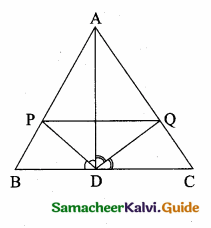But, BD = DC (D is the midpoint of BC)
Now (2) ⇒ ∴ AQQC = ADBD
From (1) and (3) We get,
∴ APPB = AQQC
Thus PQ || BC (converse of thales theorem)

Also given ∆ DEA ~ ∆ CEB
DECE = EAEB = DACB
DECE = EAEB …………(2)
From (1) and (2) we get
BEEA = EAEB ⇒ EB2 = EA2
∴ EB = EA
Substitute in (2) we get
EAEA = DACB
1 = DACB ⇒ ∴ AD = DC Hence it is proved

Tamilnadu, Samacheer, Kalvi, 10th, sslc, Maths, Solutions, Chapter 4, Algebra, unit exercise 4,

Question 1.
In the figure, if BD ⊥ AC and CE ⊥ AB , prove that
(ii) \frac { CA }{ AB } = \frac { CE }{ DB }

Solution:
(i) ∠AEC = ∠ADB = 90° ∠A is common By AA – Similarity.
Since the two triangles are similar

(ii) AEAD = ACAB = ECDB
ACAB = CEDB

Question 2.
In the given figure AB || CD || EF. If AB = 6 cm, CD = x cm, EF = 4 cm, BD = 5 cm and DE = y cm. Find x and y.

Solution:
In the given diagram ∆AEF and ∆ACD
∠AEF = ∠ACD = 90°
∠A is common
By AA – Similarity.
∴ ∆AEF ~ ∆ACD
AEAC = EFCD
AEAC = 4x
AC = AE×x4 …..(1)
In ∆EAB and ∆ECD,
∠EAB = ∠ECD = 90°
∠E is common
∆ ECD ~ ∆EAB
ECEA = EDEB = CDAB
ECEA = x6
EC = EA×x6 …….(2)
In ∆AEB; CD || AB
By Basic Proportionality Theorem
ABCD = EBED
6x = 5+yy
x = 6yy+5 (EC = x) …….(3)
Add (1) and (2) we get
AC + EC = AE×x4+x×EA6

Substitute the value of x = 2.4 in (3)
2.4 = 6yy+5
6y = 2.4y + 12
6y – 2.4y = 12 ⇒ 3.6 y = 12
y = 123.6 = 12036 = 103 = 3.3 cm
The value of x = 125 (or) 2.4 cm and y = 103(or) 3.3 cm

Question 3.
O is any point inside a triangle ABC. The bisector of ∠AOB, ∠BOC and ∠COA meet the sides AB, BC and CA in point D, E and F respectively. Show that AD × BE × CF = DB × EC × FA.
Solution:
In ∆ABC the bisector meets AB at D, BC at E and AC at F.

The angle bisector AO, BO and CO intersect at “O”.
By Cevas Theorem
ADDB × BEEC × CFAF = 1
AD × BE × CF = DB × EC × AF
Hence it is proved

Question 4.
In the figure, ABC is a triangle in which AB = AC. Points D and E are points on the side AB and AC respectively such that AD = AE. Show that the points B, C, E and D lie on a same circle.

Solution:
∠B = ∠C (Given AB = AC)
AD + DB = AE + EC
BD = EC (Given AD = AE)
DE parallel BC Since AEC is a straight line.
∠AED + ∠CED = 180°
∠CBD + ∠CED = 180°
Similarly of the opposite angles = 180°
∴ BCED is a cyclic quadrilateral

Question 5.
Two trains leave a railway station at the same time. The first train travels due west and the second train due north. The first train travels at a speed of 20 km/hr and the second train travels at 30 km/hr. After 2 hours, what is the distance between them?
Solution:
A is the position of the 1st train.
B is the position of the 2nd train.

Distance Covered in 2 hours
OA = 2 × 20 = 40 km
OB = 2 × 30 = 60 km
Distance between the train after 2 hours

Distance between
the two train = 72.11 km (or) 2013−−√ km

Question 6.
D is the mid point of side BC and AE ⊥ BC. If BC = a, AC = b, AB = c, ED = x, AD = p and AE = h, prove that
(i) b2 = p2 + ax + a24
(ii) c2 = p2 – ax + a24
(iii) a2 + c2 = 2 p2 + a22
Solution:
(i) Given ∠AED = 90°

ED = x; DC = a2
(D is the mid point of BC)
∴ EC = x + a2, BE = a2 – x
∴ In the right ∆ AED
p2 = h2 + x2
In the right ∆ AEC,
AC2 = AE2 + EC2

(ii) In the right triangle ABE,
AB2 = AE2 + BE2
c2 = h2 + (a2 – x)2
c2 = h2 + a24 + x2 – ax
c2 = h2 + x2 + 14 a2 – ax
c2 = p2 – ax + a24 (from 1)

(iii) By adding (2) and (3)
b2 + c2 = p2 + ax + a24 + p2 – ax + a24
= 2p2 + 2a24
= 2p2 + a22

Question 7.
A man whose eye-level is 2 m above the ground wishes to find the height of a tree. He places a mirror horizontally on the ground 20 m from the tree and finds that if he stands at a point C which is 4 m from the mirror B, he can see the reflection of the top of the tree. How height is the tree?
Solution:
Let the height of the tree AD be “h”.

In ∆ ACD and ∆ BCF,
∠A = ∠B = 90°
∠C is common
∆ ACD ~ ∆ BCF by AA similarity
hx = 242 = 6
h = 6x ………(1)
In ∆ ACE and ∆ ABF,
∠C = ∠B = 90°
∠A is common
∴ ∆ ACE ~ ∆ ABF
CEBF = ACAB
2x = 2420
24x = 20 × 2
x = 20×224=5×26=106
x = 53
Substitute the value of x in (1)
h = 6 × 53 = 10 m
∴ Height of the tree is 10 m

Question 8.
An emu which is 8 ft tail is standing at the foot of a pillar which is 30 ft high. It walks away from the pillar. The shadow of the emu falls beyond emu. What is the relation between the length of the shadow and the distance from the emu to the pillar?
Solution:
Let the shadow of the emu AE be “x” and BE be “y” ED || BC

By basic proportionality theorem
AEAB = EDBC
xx+y = 830
30x = 8x + 8y
22x – 8y = 0
(÷ by 2) 11x – 4y = 0
11x = 4y
x = 411 × y
x = 411 × distance from the pillar to emu
Length of = 411 × distance from the shadow the pillar to emu

Question 9.
Two circles intersect at A and B. From a point P on one of the circles lines PAC and PBD are drawn intersecting the second circle at C and D. Prove that CD is parallel to the tangent at P.
Solution:
Proof:

A and B are the points intersecting the circles. Join AB.
∠P’PB = ∠PAB (alternate segment theorem)
∠PAB + ∠BAC = 180° …(1)
(PAC is a straight line)
∠BAC + ∠BDC = 180° …(2)
From (1) and (2) we get
∠P’PB = ∠PAB = ∠BDC
P’P and DC are straight lines.
PD is a transversal alternate angles are equal.
∴ P’P || DC.

Question 10.
Let ABC be a triangle and D, E, F are points on the respective sides AB, BC, AC (or their extensions).
Let AD : DB = 5 : 3, BE : EC = 3 : 2 and AC = 21. Find the length of the line segment CF.
Solution:

ADDB = 53; BEEC = 32; AC = 21
By Ceva’s theorem

Length of the line segment CF = 6 units

Tamilnadu, Samacheer, Kalvi, 10th, sslc, Maths, Solutions, Chapter 4, Algebra, Ex 4.5,

Question 1.
If in triangles ABC and EDF, ABDE = BCFDthen they will be similar, when ……….
(1) ∠B = ∠E
(2) ∠A = ∠D
(3) ∠B = ∠D
(4) ∠A = ∠F
(3) ∠B = ∠D
Hint:

Question 2.
In ∆LMN, ∠L = 60°, ∠M = 50°. If ∆LMN ~ ∆PQR then the value of ∠R is ……………
(1) 40°
(2) 70°
(3) 30°
(4) 110°
(2) 70°
Hint:
Since ∆LMN ~ ∆PQR
∠N = ∠R
∠N = 180 – (60 + 50)
= 180 – 110°
∠N = 70° ∴ ∠R = 70°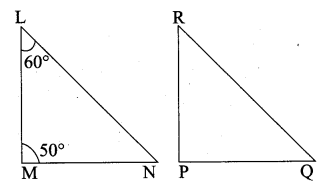Question 3.
If ∆ABC is an isosceles triangle with ∠C = 90° and AC = 5 cm, then AB is ………
(1) 2.5 cm
(2) 5 cm
(3) 10 cm
(4) 5 2–√ cm
(4) 5 2–√ cm
Hint:

AB2 = AC2 + BC2
AB2 = 52 + 52
(It is an isosceles triangle)
AB = 50−−√ = 25×2−−−−−√
AB = 5 2–√

Question 4.
In a given figure ST || QR, PS = 2 cm and SQ = 3 cm. Then the ratio of the area of ∆PQR to the area of ∆PST is …………….

(1) 25 : 4
(2) 25 : 7
(3) 25 : 11
(4) 25 : 13
(1) 25 : 4
Hint. Area of ∆PQR : Area of ∆PST
Area of ΔPQR Area of ΔPST=PQ2PS2=5222=254
Area of ∆PQR : Area of ∆PST = 25 : 4

Question 5.
The perimeters of two similar triangles ∆ABC and ∆PQR are 36 cm and 24 cm respectively. If PQ =10 cm, then the length of AB is ………….
(1) 6 23 cm
(2) 106√3
(3) 66 23 cm
(4) 15 cm
(4) 15 cm
Hint:

www.asterclasses.com

Question 6.
If in ∆ABC, DE || BC. AB = 3.6 cm, AC = 2.4 cm and AD = 2.1 cm then the length of AE is ………..
(1) 1.4 cm
(2) 1.8 cm
(3) 1.2 cm
(4) 1.05 cm
(1) 1.4 cm
Hint:

Question 7.
In a ∆ABC, AD is the bisector of ∠BAC. If AB = 8 cm, BD = 6 cm and DC = 3 cm.
The length of the side AC is ………….
(1) 6 cm
(2) 4 cm
(3) 3 cm
(4) 8 cm
(2) 4 cm
Hint:

www.asterclasses.com

Question 8.
In the adjacent figure ∠BAC = 90° and AD ⊥ BC then ………..

(1) BD.CD = BC2
(2) AB.AC = BC2
Hint:

Question 9.
Two poles of heights 6 m and 11m stand vertically on a plane ground. If the distance between their feet is 12 m, what is the distance between their tops?
(1) 13 m
(2) 14 m
(3) 15 m
(4) 12.8 m
(1) 13 m
Hint:

www.asterclasses.com

Question 10.
In the given figure, PR = 26 cm, QR = 24 cm, ∠PAQ = 90°, PA = 6 cm and QA = 8 cm. Find ∠PQR.

(1) 80°
(2) 85°
(3) 75°
(4) 90°
(4) 90°
Hint.
PR = 26 cm, QR = 24 cm, ∠PAQ = 90°
In the ∆PQR,

In the right ∆ APQ
PQ2 = PA2 + AQ2
= 62 + 82
= 36 + 64 = 100
PQ = 100−−−√ = 10
∆ PQR is a right angled triangle at Q. Since
PR2 = PQ2 + QR2
∠PQR = 90°

Question 11.
A tangent is perpendicular to the radius at the
(1) centre
(2) point of contact
(3) infinity
(4) chord
Solution:
(2) point of contact

Question 12.
How many tangents can be drawn to the circle from an exterior point?
(1) one
(2) two
(3) infinite
(4) zero
(2) two

Question 13.
The two tangents from an external points P to a circle with centre at O are PA and PB.
If ∠APB = 70° then the value of ∠AOB is ……….
(1) 100°
(2) 110°
(3) 120°
(4) 130°
(2) 110°
Hint.

∠OAP = 90°
∠APO = 35°
∠AOP = 180 – (90 + 35)
= 180 – 125 = 55
∠AOB = 2 × 55 = 110°

www.asterclasses.com

Question 14.
In figure CP and CQ are tangents to a circle with centre at 0. ARB is another tangent touching the circle at R. If CP = 11 cm and BC = 7 cm, then the length of BR is …….

(1) 6 cm
(2) 5 cm
(3) 8 cm
(4) 4 cm
(4) 4 cm
Hint.
BQ = BR = 4 cm (tangent of the circle)
PC = QC = 11 cm (tangent of the circle)
QC = 11 cm
QB + BC = 11
QB + 7 = 11
QB = 11 – 7 = 4 cm
BR = BQ = 4 cm

Question 15.
In figure if PR is tangent to the circle at P and O is the centre of the circle, then ∠POQ is ………….

(1) 120°
(2) 100°
(3) 110°
(4) 90°
(1) 120°
Hint.
Since PR is tangent of the circle.
∠QPR = 90°
∠OPQ = 90° – 60° = 30°
∠OQB = 30°
In ∆OPQ
∠P + ∠Q + ∠O = 180°
30 + 30° + ∠O = 180°
(OP and OQ are equal radius)
∠O = 180° – 60° = 120°

Tamilnadu, Samacheer, Kalvi, 10th, sslc, Maths, Solutions, Chapter 4, Algebra, Ex 4.4,

Question 1.
The length of the tangent to a circle from a point P, which is 25 cm away from the centre is 24 cm. What is the radius of the circle?
Solution:
Let the radius AB be r. In the right ∆ ABO,
OB2 = OA2 + AB2
252 = 242 + r2

252 – 242 = r2
(25 + 24) (25 – 24) = r2
r = 49−−√ =7
Radius of the circle = 7 cm

Question 2.
∆ LMN is a right angled triangle with ∠L = 90°. A circle is inscribed in it. The lengths of the sides containing the right angle are 6 cm and 8 cm. Find the radius of the circle.
Solution:
LN = 6; ML = 8. In the right ∆ LMN,
MN2 = LN2 + LM2
= 62 + 82 = 36 + 64 = 100
MN = 100−−−√ = 10

Question 3.
A circle is inscribed in ∆ ABC having sides 8 cm, 10 cm and 12 cm as shown in figure, Find AD, BE and CF.

Solution:
AD = AF = x (tangent of the circle)
BD = BE = y (tangent of the circle)
CE = CF = z (tangent of the circle)
x + y = 12 ……(1)
BC = BE + EC
y + z= 8 …….(2)
AC = AF + FC
x + z = 10 ……(3)
2x + 2y + 2z = 12 + 8 + 10
x + y + z = 302 = 15 …….(4)
By x + y = 12 in (4)
z = 3
y + z = 8 in (4)
x = 7
x + z = 10 in (4)
y = 5
AD = 7 cm; BE = 5 cm and CF = 3 cm

Question 4.
PQ is a tangent drawn from a point P to a circle with centre O and QOR is a diameter of the circle such that ∠POR = 120° . Find ∠OPQ.
Solution:
∠POQ = 180° – 120° = 60°
In ∆OPQ, we know

∠POQ + ∠OQP + ∠OPQ = 180°
(Sum of the angles of a ∆ is 180°)
60° + 90° + ∠OPQ = 180°
∠OPQ = 180° – 150° = 30°
∠OPQ = 30°

Question 5.
A tangent ST to a circle touches it at B. AB is a chord such that ∠ABT = 65°. Find ∠AOB, where “O” is the centre of the circle.
Solution:
Given ∠ABT = 65°
∠OBT = 90°(TB is the tangent of the circle)
∠ABO = 90° – 65° = 25°
∠ABO + ∠BOA + ∠OAB = 180°
25° + x + 25° = 180° (Sum of the angles of a ∆)

(OA and OB are the radius of the circle.
∴ ∠ABO = ∠BAO = 25°
x + 50 = 180°
x = 180° – 50° = 130°
∴ ∠BOA = 130°

Question 6.
In figure, O is the centre of the circle with radius 5 cm. T is a point such that OT = 13 cm and OT intersects the circle E, if AB is the tangent to the circle at E, find the length of AB.

Solution:
In the right ∆ OTP,
PT2 = OT2 – OP2
= 132 – 52
= 169 – 25
= 144
PT = 144−−−√ = 12 cm
Since lengths of tangent drawn from a point to circle are equal.
∴ AP = AE = x
AT = PT – AP
= (12 – x) cm
Since AB is the tangent to the circle E.
∴ OE ⊥ AB
∠OEA = 90°
∠AET = 90°
In ∆AET, AT2 = AE2 + ET2
In the right triangle AET,
AT2 = AE2 + ET2
(12 – x)2 = x2 + (13 – 5)2
144 – 24x + x2 = x2 + 64
24x = 80 ⇒ x = 8024 = 206 = 103
BE = 103 cm
AB = AE + BE
= 103 + 103 = 203
Lenght of AB = 203 cm

Question 7.
In two concentric circles, a chord of length 16 cm of larger circle becomes a tangent to the smaller circle whose radius is 6 cm. Find the radius of the larger circle.
Solution:
Here AP = PB = 8 cm
In ∆OPA,
OA2 = OP2 + AP2

= 62 + 82
= 36 + 64
= 100
OA = 100−−−√ = 10 cm
Radius of the larger circle = 10 cm

www.asterclasses.com

Question 8.
Two circles with centres O and O’ of radii 3 cm and 4 cm, respectively intersect at two points P and Q, such that OP and O’ P are tangents to the two circles. Find the length of the common chord PQ.
Solution:
In ∆ OO’P
(O’O)2 = OP2 + O’P2
= 32 + 42

= 9 + 16
(OO’)2 = 25
∴ OO’ = 5cm
Since the line joining the centres of two intersecting circles is perpendicular bisector of their common chord.
OR ⊥ PQ and PR = RQ
Let OR be x, then O’R = 5 – x again Let PR = RQ = y cm
In ∆ ORP, OP2 = OR2 + PR2
9 = x2 + y2 …(1)
In ∆ O’RP, O’P2 = O’R2 + PR2
16 = (5 – x)2 + y2
16 = 25 + x2 – 10x + y2
16 = x2 + y2 + 25 – 10x
16 = 9 + 25 – 10x (from 1)
16 = 34 – 10x
10x = 34 – 16 = 18
x = 1810 = 1.8 cm
Substitute the value of x = 1.8 in (1)
9 = (1.8)2 + y2
y2 = 9 – 3.24
y2 = 5.76
y = 5.76−−−−√ = 2.4 cm
Hence PQ = 2 (2.4) = 4.8 cm
Length of the common chord PQ = 4.8 cm

Question 9.
Show that the angle bisectors of a triangle are concurrent.
Solution:
Given: ABC is a triangle. AD, BE and CF are the angle bisector of ∠A, ∠B, and ∠C.
To Prove: Bisector AD, BE and CF intersect
Proof: The angle bisectors AD and BE meet at O. Assume CF does not pass through O. By angle bisector theorem.
AD is the angle bisector of ∠A
BDDC = ABAC …..(1)
BE is the angle bisector of ∠BCEEA = BCAB …….(2)
CF is the angle bisector ∠C
AFFB = ACBC …….(3)
Multiply (1) (2) and (3)
BDDC × CEEA × AFFB = ABAC × BCAB × ACBC
So by Ceva’s theorem.
The bisector AD, BE and CF are concurrent.

Question 10.
In ∆ABC , with ∠B = 90° , BC = 6 cm and AB = 8 cm, D is a point on AC such that AD = 2 cm and E is the midpoint of AB. Join D to E and extend it to meet at F. Find BF.
Solution:
Consider ∆ABC. Then D, E and F are respective points on the sides AC, AB and BC.
By construction D, E, F are collinear.
By Menelaus’ theorem AEEB × BFFC × CDDA = 1 ……(1)
AD = 2 cm; AE = EB = 4 cm; BC = 6 cm; FC = FB + BC = x + 6
In ∆ABC, By Pythagoras theorem.

AC2= AB2 + BC2
AC2 = 82 + 62 = 64 + 36 = 100
AC = 100−−−√ = 10
= 10 – 2 = 8 cm
Substituting the values in (1) we get
44 × xx+6 × 82 = 1
xx+6 × 4 = 1
4x = x + 6
3x = 6 ⇒ x = 63 = 2
The value of BF = 2 cm

Question 11.
An artist has created a triangular stained glass window and has one strip of small length left before completing the window. She needs to figure out the length of left out portion based on the lengths of the other sides as shown in the figure.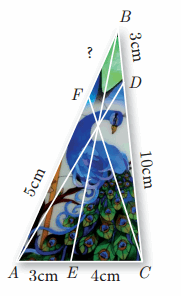Solution:
Given that AE = 3 cm, EC = 4 cm, CD = 10 cm, DB = 3 cm, AF = 5 cm.
Let FB be x
Using Ceva’s theorem we have
AEEC × CDDB × BFAF = 1
34 × 103 × x5 = 1
2×4 = 1
2x = 4 ⇒ x = 42 = 2
The value of BF = 2

Question 12.
Draw a tangent at any point R on the circle of radius 3.4 cm and centre at P ?Steps of construction:

1. Draw a circle with centre “O” of radius 3.4 cm.
2. Take a point P on the circle Join OP.
3. Draw a perpendicular line TT’ to OP which passes through P.
4. TT’ is the required tangent.

www.asterclasses.com

Question 13.
Draw a circle of radius 4.5 cm. Take a point on the circle. Draw the tangent at that point using the alternate segment theorem.
Radius of the circle = 4.5 cm

1. With O as centre, draw a circle of radius 4.5 cm.
2. Take a point L on the circle. Through L draw any chord LM.
3. Take a point M distinct from L and N on the circle, so that L, M, N are in anti-clockwise direction. Join LN and NM.
4. Through “L” draw tangent TT’such that ∠TLM = ∠MNL
5. TT’ is the required tangent.

Question 14.
Draw the two tangents from a point which is 10 cm away from the centre of a circle of radius 5 cm. Also, measure the lengths of the tangents.
Radius = 5 cm; Distance = 10 cm

1. With O as centre, draw a circle of radius 5 cm.
2. Draw a line OP =10 cm.
3. Draw a perpendicular bisector of OP, which cuts OP at M.
4. With M as centre and MO as radius draw a circle which cuts previous circle at A and B.
5. Join AP and BP. AP and BP are the required tangents.

www.asterclasses.com

Verification: In the right ∆ OAP
PA2 = OP2 – OA2
= 102 – 52 = 100−25−−−−−−−√ = 75−−√ = 8.7 cm
Lenght of the tangent is = 8.7 cm

1. With O as centre, draw a circle of radius 4 cm.
2. Draw a line OP = 11 cm.
3. Draw a perpendicular bisector of OP, which cuts OP at M.
4. With M as centre and MO as radius, draw a circle which cuts previous circle A and B.
5. Join AP and BP. AP and BP are the required tangents.

This the length of the tangents PA = PB = 10.2 cm
Verification: In the right angle triangle OAP
PA2 = OP2 – OA2
= 112 – 42 = 121 – 16 = 105
PA = 105−−−√ = 10.2 cm
Length of the tangents = 10.2 cm

Question 16.
Draw the two tangents from a point which is 5 cm away from the centre of a circle of diameter 6 cm. Also, measure the lengths of the tangents.
Radius = 3cm; Distance = 5cm.

1. With O as centre, draw a circle of radius 3 cm.
2. Draw a line OP = 5 cm.
3. Draw a perpendicular bisector of OP, which cuts OP at M.
4. With M as centre and MO as radius draw a circle which cuts previous circles at A and B.
5. Join AP and BP, AP and BP are the required tangents.

The length of the tangent PA = PB = 4 cm
Verification: In the right angle triangle OAP
PA2 = OP2 – OA2
= 52 – 32
= 25 – 9
= 16 PA
= 16−−√ = 4 cm
Length of the tangent = 4 cm

Question 17.
Draw a tangent to the circle from the point P having radius 3.6 cm, and centre at O. Point P is at a distance 7.2 cm from the centre.
Radius = 3.6; Distance = 7.2 cm.

Steps of construction:

1. With O as centre, draw a circle of radius 3.6 cm.
2. Draw a line OP = 7.2 cm.
3. Draw a perpendicular bisector of OP which cuts OP at M.
4. With M as centre and MO as radius draw a circle which cuts the previous circle at A and B.
5. Join AP and BP, AP and BP are the required tangents.

Length of the tangents PA = PB = 6.26 cm
Verification: In the right triangle ∆OAP
PA2 = OP2 – OA2
= 7.22 – 3.62 =(7.2 + 3.6) (7.2 – 3.6)
PA2 = 10.8 × 3.6 = 38.88−−−−√
PA = 6.2 cm
Length of the tangent = 6.2 cm

Tamilnadu, Samacheer, Kalvi, 10th, sslc, Maths, Solutions, Chapter 4, Algebra, Ex 4.3,

Question 1.
A man goes 18 m due east and then 24 m due north. Find the distance of his current position from the starting point?
Solution:
Let the initial position of the man be “O” and his final
position be “B”.
By Pythagoras theorem
In the right ∆ OAB,

OB2 = OA2 + AB2
= 182 + 242
= 324 + 576 = 900
OB = 900−−−√ = 30
The distance of his current position is 30 m

Question 2.
There are two paths that one can choose to go from Sarah’s house to James house. One way is to take C street, and the other way requires to take A street and then B street. How much shorter is the direct path along C street? (Using figure).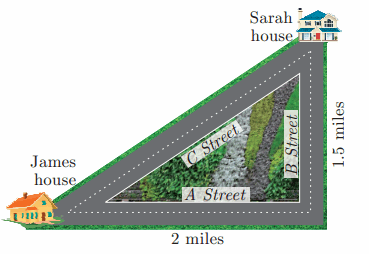Solution:
Distance between Sarah House and James House using “C street”.
AC2 = AB2 + BC2
= 22 + 1.52
= 4 + 2.25 = 6.25
AC = 6.25−−−−√
AC = 2.5 miles

Distance covered by using “A Street” and “B Street”
= (2 + 1.5) miles = 3.5 miles
Difference in distance = 3.5 miles – 2.5 miles = 1 mile

Question 3.
To get from point A to point B you must avoid walking through a pond. You must walk 34 m south and 41 m east. To the nearest meter, how many meters would be saved if it were possible to make a way through the pond?
Solution:
In the right ∆ABC,
By Pythagoras theorem
AC2= AB2 + BC2 = 342 + 412
= 1156 + 1681 = 2837
AC = 2837−−−−√
= 53.26 m

Through A one must walk (34m + 41m) 75 m to reach C.
The difference in Distance = 75 – 53.26
= 21.74 m

Question 4.
In the rectangle WXYZ, XY + YZ = 17 cm, and XZ + YW = 26 cm.
Calculate the length and breadth of the rectangle?

Solution:
Let the length of the rectangle be “a” and the breadth of the rectangle be “b”.
XY + YZ = 17 cm
b + a = 17 …….. (1)
In the right ∆ WXZ,
XZ2 = WX2 + WZ2
(XZ)2 = a2 + b2XZ = a2+b2−−−−−−√
Similarly WY = a2+b2−−−−−−√ ⇒ XZ + WY = 26
2 a2+b2−−−−−−√ = 26 ⇒ a2+b2−−−−−−√ = 13
Squaring on both sides
a2 + b2 = 169
(a + b)2 – 2ab = 169
172 – 2ab = 169 ⇒ 289 – 169 = 2 ab
120 = 2 ab ⇒ ∴ ab = 60
a = 60b ….. (2)
Substituting the value of a = 60b in (1)
60b + b = 17
b2 – 17b + 60 = 0
(b – 2) (b – 5) = 0
b = 12 or b = 5If b = 12 ⇒ a = 5
If b = 6 ⇒ a = 12
Lenght = 12 m and breadth = 5 m

Question 5.
The hypotenuse of a right triangle is 6 m more than twice of the shortest side. If the third side is 2 m less than the hypotenuse, find the sides of the triangle.
Solution:
Let the shortest side of the right ∆ be x.
∴ Hypotenuse = 6 + 2x
Third side = 2x + 6 – 2
= 2x + 4

In the right triangle ABC,
AC2 = AB2 + BC2
(2x + 6)2 = x2 + (2x + 4)2
4x2 + 36 + 24x = x2 + 4x2 + 16 + 16x
0 = x2 – 24x + 16x – 36 + 16
∴ x2 – 8x – 20 = 0
(x – 10) (x + 2) = 0
x – 10 = 0 or x + 2 = 0x = 10 or x = -2 (Negative value will be omitted)
The side AB = 10 m
The side BC = 2 (10) + 4 = 24 m
Hypotenuse AC = 2(10) + 6 = 26 m

Question 6.
5 m long ladder is placed leaning towards a vertical wall such that it reaches the wall at a point 4m high. If the foot of the ladder is moved 1.6 m towards the wall, then find the distance by which the top of the ladder would slide upwards on the wall.
Solution:
“C” is the position of the foot of the ladder “A” is the position of the top of the ladder.
In the right ∆ABC,
BC2 = AC2 – AB2 = 52 – 42
= 25 – 16 = 9
BC = 9–√ = 3m.
When the foot of the ladder moved 1.6 m toward the wall.
The distance between the foot of the ladder to the ground is
BE = 3 – 1.6 m
= 1.4 m

Let the distance moved upward on the wall be “h” m
The ladder touch the wall at (4 + h) M
In the right triangle BED,
ED2 = AB2 + BE2
52 = (4 + h)2 + (1.4)2
25 – 1.96= (4 + h)2
∴ 4 + h = 23.04−−−−√
4 + h = 4. 8 m
h = 4.8 – 4
= 0.8 m
Distance moved upward on the wall = 0.8 m

Question 7.
The perpendicular PS on the base QR of a ∆PQR intersects QR at S, such that QS = 3 SR. Prove that 2PQ2 = 2PR2 + QR2.
Solution:
Given QS = 3SR
QR = QS + SR
= 3SR + SR = 4SR
SR = 14 QR …..(1)
QS = 3SR
SR = QS3 ……..(2)

From (1) and (2) we get
14 QR = QS3
∴ QS = 34 QR ………(3)
In the right ∆ PQS,
PQ2 = PS2 + QS2 ……….(4)
Similarly in ∆ PSR
PR2 = PS2 + SR2 ………..(5)
Subtract (4) and (5)
PQ2 – PR2 = PS2 + QS2 – PS2 – SR2
= QS2 – SR2

PQ2 – PR2 = 12 QR2
2PQ2 – 2PR2 = QR2
2PQ2 = 2PR2 + QR2
Hence the proved.

Question 8.
In the adjacent figure, ABC is a right angled triangle with right angle at B and points D, E trisect BC. Prove that 8AE2 = 3AC2 + 5AD2.
Solution:
Since the Points D, E trisect BC.
BD = DE = CE
Let BD = DE = CE = x
BE = 2x and BC = 3x

In the right ∆ABD,
In the right ∆ABE,
AE2 = AB2 + 2BE2
AE2 = AB2 + 4X2 ………..(2) (BE = 2x)
In the right ∆ABC
AC2 = AB2 + BC2
AC2 = AB2 + 9x2 …………… (3) (BC = 3x)
= 3[AB2 + 9x2] + 5 [AB2 + x2] [From (1) and (3)]
= 3AB2 + 27x2 + 5AB2 + 5x2
= 8AB2 + 32x2
= 8 (AB2 + 4 x2)
= 8AE2 [From (2)]
= R.H.S.
∴ 8AE2 = 3AC2 + 5AD2

#### VISITORS COUNTUsers Today : 40Total Users : 364533Views Today : 361Total views : 1260268